Light pressure fog caused by Maxwell-Bartoli formula

Guan Keying

Department of Mathematics, School of Science, Beijing Jiaotong University

Email: keying.guan@gmail.com

Abstract: It is proven that the so-called radiation pressure defined by the Maxwell-Bartoli formula is not mechanical pressure at all, causing confusion in dimensions and quantities, and has had an extremely negative impact on physics so far. In addition, based on the special theory of relativity, electromagnetic radiation theory, and photon theory, it is proven the following rule related to radiation pressure: when light (electromagnetic radiation) hits ordinary matter with non-zero static mass, it must immediately cause changes in the physical state of the incedent radiation, or of the irradiated substance or of both of them.

I Background

From the beginning of April 2021, the author gradually transitioned to a series of discussions on the light pressure. In the light pressure discussion stage, it was found that there were serious problems in the theory, the experiments, and the popular interpretation of the light pressure. The author noticed that since Maxwell established the electromagnetic wave equation in the nineteenth century, leading physicists have given different light pressure expressions and experiments based on different considerations. But judging from the pressure expressed by the now generally recognized Maxwell-Bartoli formula, there is a denominator in the formula, namely the speed of light, which brings a series of confusion. To clarify this issue, the author traced the source to this problem. The author found that this formula had not any reasonably derivation process and found the wrong method of ​​Maxwell and Bartoli in estimating the so-called solar radiation pressure. During the exploration process, the author also discovered a universal rule of electromagnetic radiation interacting with matter of non-zero static mass, which can explain a series of related phenomena.

II Maxwell-Bartoli pressure is not true radiation pressure

If light beams or electromagnetic radiations are regarded as flowing matter, then the main difference between this kind of matter and ordinary matter is that it has no static mass, while ordinary matter has a static mass. This determines the essential difference between the effect of electromagnetic radiation on ordinary substances and the interactive effect among ordinary substances.

Will light exert pressure on ordinary substances? In fact, this is an old topic. As early as 1619, the famous German astronomer Johannes Kepler imagined that the tail of a comet was caused by the pressure of sunlight. Since then, many famous scholars have participated in research and discussion.

The electromagnetic field theory gradually matured in the middle and late 19th century. The great British (Scottish) mathematical physicist James Clerk Maxwell established the electromagnetic field motion equation in 1865  , and derived the expression of the energy density of electromagnetic radiation, etc., so that electromagnetic Radiation is seen as the theoretical basis of actual matter. He pointed out in 1873 based on the principle of electromagnetics ,  that if light is an electromagnetic phenomenon, then the pressure should be generated by the absorption or reflection of the light beam. After discussing the equations involved, he said: “Therefore, in the propagation medium of the wave, the pressure in the direction perpendicular to the wave is numerically equal to the pressure corresponding to the energy per unit volume.”  Maxwell also calculated that the sun light pressure exerted on the illuminated surface of the earth, and the following narrative is added:

“Concentrated light through the electric lamp may obtain greater radiant energy. Such light falls on a small metal disk and is suspended in a vacuum, which may produce observable mechanical effects.”

However, based on a series of clues, the author believes that Maxwell’s calculated solar radiation pressure is 0.00000041 kilogram-force per square meter, which seems too small. To find out the reason, the author searched his original work. It is a pity that the author has not found his 1873 English original . Fortunately, the author found the 1884 German version of this work , so that the author can understand his calculation process and thoughts. The German text of this part is as follows:

§793. Nimmt man an, dass kraftiges Sonnenlicht an einem Quadratmeter in der Secunde 124,1 Kilogrammeter Energie entwickelt, so warden in einem Kubikmeter Sonnenstrahlen 124/300000000 oder 0,00000041 Kilo-gram Drmeter Energie entachreuck sein. eine zur Fortpflanzungsrichtung der Sonnenstrahlen senkrechte Flache pro Quadratmeter erleidet, 0,00000041 Kilogrammeter betragen.

English translation is

§793. Assuming that powerful sunlight develops 124.1 kilograms of energy in one square meter per second, one cubic meter of sun rays would contain 124/300000000 or 0.00000041 kilograms of energy. According to this, the mean pressure per square meter that a surface perpendicular to the direction of propagation of the sun's rays suffers would be 0.00000041 kilograms.

Now, consider what he made the statement below

powerful sunlight develops 124.1 kilograms of energy in one square meter per second, one cubic meter of sun rays

The author think this statememt is somewhat flawed in terms of dimensions. The author will try to make this statement clearer.

It can be inferred that this expression is based on the solar constant measured by the French physicist Claude Pouillet, that is, the intensity of solar radiation received by the ground is 1.228 kilowatts per square meter. Converting this value into mechanical work, then converting it into work done by mechanical force.  is somewhat flawed in terms of dimensions. The author will make a clearer statement of this statement.

In the blog post <The standard sunlight pressure on the ground is equivalent to 1310 Pa>

http://blog.sciencenet.cn/home.php?mod=space&uid=553379&do=blog&id=1286877

the author proved that the radiation intensity of n (is a pure number) watt per second per square meter is equivalent to applying the constant force，n Newton (N) to a uniform material surface with a standard area mass density, that is, one kilogram per square meter, and pushing the surface to move forward one meter within n fraction of a second, or orquivalently proved that the radiation intensity of n watt per second per square meter is equivalent to applying a constant force， n Newton (N) to a uniform material surface with a area mass density, n kilogram per square meter, and pushing the surface to move forward one meter in a second. So, the surface will feel a pressure of n Newton per square meter.

Note that 1 kilonewton is equivalent to 102.0 kgf. Therefore, the solar radiation intensity measured by Pouillet is 1.228 kW/m2/sec, which is theoretically equivalent to a radiation pressure of 125.6 kgf per square meter.  This value is basically the same as the 124.1 kilograms used by Maxwell. Therefore, the above statement of Maxwell should mean that

powerful sunlight develops 124.1 kilograms pressue force in one square meter .

For the convenience of the further discussion, the radiation pressure, such as 125.6, or 124.1 kilogram force per square meter calculated by the above method, will be called the “equivalent radiation pressure”. It is not difficult to see that the “equivalent radiation pressure of sunlight” is approximately one hundredth of the atmospheric pressure on the earth's surface. The author think this theoretical radiation pressure is reasonable, though it is cannot be measured in practice (see the Part III of this article).

If Maxwell really accepted the pressure of 124.1 kilograms of force per square meter as the theoretical radiation pressure of sunlight, the research on radiation pressure would be on the right track. However, Maxwell divided this theoretical pressure by a pure number of 300 million and got an incredibly small pressure value, i.e.,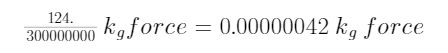for each square meter. Interpreting this quantity as light pressure, no matter how you think about it, you will find that this is totally unreasonable.

If Maxwell's original intention were to divide the intensity of the radiation by the speed of light 300,000,000 m/s, this does have important physical meaning. Based on the theory of relativity and quantum mechanics, this quantity can be interpreted as the momentum flow density of electromagnetic radiation acting on every square meter of surface. Unfortunately, at that time there was not the famous general energy expression E=Mc2 given by Einstein, and Maxwell could not have realized this.

Even more sad: In 1876, the Italian physicist, Adolfo Bartoli, independently of Maxwell, thought about the existence of radiation pressure from a thermodynamic point of view. He believes that the radiation temperature of an object can be raised by moving the mirror to reflect its light, so energy can be transmitted from a colder object to a hotter object. To avoid violating the second law of thermodynamics, light must exert pressure on the mirror. He gave a radiation pressure formula,(1)

where v is the speed of light, and E is interpreted as in the original Italian “l’equivalente meccanico del calore”, translated into English as the mechanical equivalent of heat, Q  is the radiation Heat received by the surface of a square meter within one second.

Since formula (1) has the denominator of the speed of light v, it is like Maxwell, which greatly dilutes the heat or “equivalent light pressure”. To understand Bartoli’s argumentation process, the author found the unclear copy of the main 4 pages (195-198) of Bartoli’s original article  through Google, translated it into English through Google, and finally found that Bartoli did not give any mathematical proof of the formula, (1) can only be understood as a proposition of his own imagination.

The symbols used in formula (1) are not familiar to modern people. In 1901, the Russian physicist Peter Lebedew in his famous paper  rewritten formula (1) into a more understandable form(1')

where E is the total energy passing through a unit area per unit time, V is the speed of light, andis the reflection coefficient. Lebedew pointed out that this pressure is exceedingly small in his paper, because he recognized the calculated values on the sun light pressure of Maxwell and Bartoli, so he called the pressure (1') as "Maxwell-Bartoli" pressure. Clearly, he did not prove this formula theoretically.

If (1') is considered correct, then when the illuminated object is an absolute black body, i.e., the reflection coefficient is equal to zero, then (1') is simplified to(1'.1)

The above formula is used by many scholars as the theoretical basis for the small light pressure.

In order to study the absurdity of this formula, a modern form of (1'.1) will be given below.

Firstly, the modern notation of the speed of light is c.

For simple, assume that the radiation is propagation in the vacuum.

The British physicist John Henri Poynting found  an important vector of the electromagnetic radiation (now is called as the Poynting vector)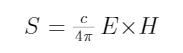(2)

where E and H are the electric field strength and the magnetic field strength, respectively. It represents the total energy flow passing through a unit area per unit time, and the light speed c in (2) should be regarded as a non-directional quantity in this equality.

S represents the total energy flow passing through a unit area per unit time.

Note that in his time, Poynting used an old and complicated form to describe this vector (see the equation (7) of page 348 in ). The present notations used by the present author were from the famous monograph <The classic field>  by the physicist L. Landau of the former Soviet Union,

For the further description, let <S> represent the non-directed total energy contained in S. It is clearly that S or <S> is equivalent to the quantity E in (1’) and (1’1). It depends on whether you regard this part of energy as directional.

Let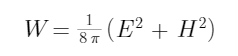(3)

represent the energy density of the radiation in a cubit meter. The conservation law of energy in electromagnetic radiation proved by Poynting is equivalent to that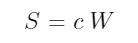(4)

Since S is a vector, the speed c on the right side of the equation must be regarded as a vector in the same direction as S.

When the light beam illuminates the surface of an object perpendicularly and creates pressure on the surface of the object, the direction of the pressure is along the propagation direction of the radiation. So, the modern expression of the radiation pressure (1'.1) must be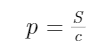(5)

where the speed of light c has no direction.

Note that the formula (5) is inconsistence with the formula appeared in Wikipedia:

According to the theory of special relativity, the electromagnetic radiation has no static mass. Nevertheless, the total energy contained in S can still be expressed as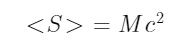(6)

where M should be understood as the moving mass of this part of electromagnetic radiation, then just like ordinary matter, the momentum P of this part of electromagnetic radiation is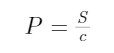（7）

where the speed of light c has no direction either.

From equations (5) and (7), the following absurd equation is obtained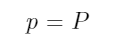(!)

Since equation (7) is an expression with actual physical meaning, it can be seen that the so-called Maxwell-Bartoli pressure is not pressure at all, but the momentum per square meter of electromagnetic radiation transferred to the surface of the material, that is, momentum density.

In fact, in classical Newtonian mechanics or relativistic mechanics, the force F is related to the momentum P as follows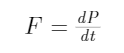Therefore, pressure and momentum are quite different in dimension and quantity.

More seriously, due to the absurd formula (!), many famous physics monographs and physics textbooks have been affected. They confuse pressure and momentum, and even use the same letter p to represent these two different physical quantities. This is commonly used in physics research (especially in the fields of thermodynamics and statistical physics) and education, which can cause serious problems!

The author hereby strongly recommends that the physics community take the problem found in this article seriously.

The author refers to the above formula (!) as "light pressure haze".

III The universal rule of interaction between light and ordinary matter

In the aforementioned research blog post <The standard sunlight pressure on the ground is equivalent to 1310 Pa>, using the mechanical power equivalent of Poynting vector S, the author derives an "equivalent pressure" formula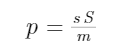(8)

where the mechanical power is in watts and the pressure is in Pa.

It will be seen that this equivalent light pressure is impossible to achieve in the real world.

In fact, in the 19th century, the research of French physicists, Nicolas Léonard Sadi Carnot (Nicolas Carnot), Benoît Paul émile Clapeyron (Emile Clapeyron), and the developed second law of thermodynamics show that: this heat “equivalent mechanical pressure” is impossible to achieve in practice, steam engine is an example.

Based on modern relativity and quantum mechanics, the author proves theoretically that it is impossible to achieve the "equivalent pressure" of radiation in realization.

Study a basic model. Along the x-axis, let a photon γ with frequency

Based on modern relativity and quantum mechanics, the author proves theoretically that it is impossible to achieve the "equivalent pressure" of radiation in realization.

Study a basic model. Along the x-axis, let a photon γ with frequency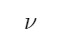shoots directly to an isolated mass point q which has a static mass m, and let the mass point q were staying in static state initially on the x-axis. Assuming that the particle is like an absolute black body, it will absorb the photon and receive all the energy and momentum of the photon. If no other matter is produced at this time, the mass point q should start to move along the x-axis, otherwise the law of conservation of energy momentum will be violated. According to the conservation of energy(9)

where h is the Planck constant,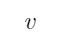is the velocity of the mass point q along the x-axis after the photon is obsorbed.

The conservation of momentum is(10)

where λ= c/ν is the wavelength of the photo γ. Due to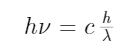(11)

and so(12)

It is not difficult to see that for any reasonable speed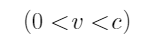, equation (12) is impossible to hold.

It is still possible that after absorbing the incident photon γ, the original particle q becomes a new particle q', and its static mass mq'. In this case, (9) should be rewritten as(9')

where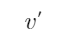is the velocity of the new mass point q’and (12) should be(12')

From (9’) and (12’), the velocityand the mass mq' can be found as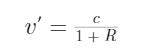(13)

and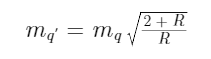(14)

where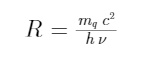It is easy to see that the speedis reasonable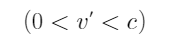and the new mass mq' is reasonably larger than the original mass m. This means the original mass point q has undergone a change in the state of matter and has become a totally new mass point q’, which received the energies and moments of both the mass point q and the incident photon γ. Normal atoms becoming excited atoms after absorbing appropriate photons may be an example of this change in the state of matter.

The remaining possibility can only be: When the stationary mass point q absorbs the incident photon γ, it will be immediately decomposed into separate parts sharing the energies and momentums of both the incident photon and original mass point. Some of them may have static mass, some of them may have not static mass. It is possible that one part of them may still the original mass point q. In this case, this mass point q cannot obtain all the energy and momentum of the incident photon γ. The Compton scattering and photoelectric effect are all examples of this possibility.

If the original particle q starts to move at a constant speed along the x-axis, then the coordinate system can be changed to a reference system that moves with it, but the frequency and wavelength of the incident photon need to be transformed accordingly. In this case, after the original particle q absorbs the incident photon γ, the above-mentioned changes in the state of matter should still occur.

In general, since ordinary materials are generally composed of elementary particles with non-zero static mass, and the light beam is a collection of photons, the above conclusion can be summarized as

When a light beam illuminates a common material, at least one of the incident light beam and the irradiated material will immediately undergo a certain state change. For examples:

1) Together they become a new material with the energy and momentum of the incident beam and the original irradiated material.

2) Through the change of the state of matter, they become at least two independent materials (some of which may be new electromagnetic radiation), sharing the total energy and momentum of the original matter and incident light. It is possible that one of them is still the original ordinary material.

What needs to be explained here is that in mechanics, the concept of force is only used to describe the motion of ordinary matter without state change, and the concept of pressure cannot be used to describe the process of state change.

When the light beam hits the surface of the ordinary material, the actual light pressure can only be measured when the original material has no state change. According to the general law derived above, it is obvious that the measured light pressure must be less than the "equivalent pressure of the incident light beam".

According to this law, ordinary matter will produce "diffuse reflection" with its own color when irradiated by light. This phenomenon became the basis for mankind to observe the material world.

The light can cause chemical changes in substances. The strong light beams can destroy, ignite, or melt some hard substances. It is even possible that a strong photon can trigger nuclear fission. All these possibilities are manifestations of this law.

Please note that the total reflection of light by a metal mirror is not a direct result of this law, because it does not clearly satisfy the law of conservation of energy and momentum. It must be a very complex state change process of related materials. This article does not discuss this issue because it is too complicated.

IV Light pressure measurement history

In 1873, the famous British chemist and physicist, William Crookes, developed a special radiometer (Crookes' Radiometer) while studying infrared radiation and element thallium . It consists of four leaves, each of which is black on one side and silver on the other side. They are mounted on the arm of the rotor, and the arm of the rotor is balanced on the vertical support so that there is little friction when it rotates. The structure is installed in a transparent glass cavity, the glass cavity has been pumped to a high degree of vacuum, but not a perfect vacuum.

When sunlight falls on the radiometer, the leave system rotates, and the black surface is obviously pushed away by the light. Crookes initially thought that this indicated that the light radiation pressure on the black blades was turning the blades, just like the water in a water mill. James Clerk Maxwell cited the paper he reported on the device and accepted Crookes' explanation. Maxwell seemed pleased to see that his electromagnetic theory predicted the effects of radiation pressure.

Using this radiometer in 1877, the light pressure measured by F. Zöllner was sometimes 100,000 times larger than the estimate (1) or (1') 

At that time, the theory of electromagnetic radiation was not perfect, and modern relativity and quantum mechanics had not yet been established. People abandon the direct explanation of the real light pressure, and try to explain the rotation phenomenon of the radiometer blade in other ways. At that time, various blunt explanations could only be put forward based on known thermodynamics, fluid mechanics and classical statistical physics, so most of these explanations were not accepted by physicists at the time. For example, Maxwell only thinks that the Irish scientist Osborne Reynolds, who has made a significant contribution to fluid mechanics, has some truth, but he also criticized his mathematical treatment , .

The author believes that any explanation of the Crooks radiometer at that time could not be adopted. The reason is simple, because they are all based on the misunderstanding of the light pressure measured by F. Zöllner and the misunderstanding of the formula (1) or (1'). In fact, in the major works of modern thermals, statistical physics, or aerodynamics, we have not seen any such theory or mathematical model that can successfully explain the Crookes radiometer.

In 1901, P. Lebedew and American physicists E.F. Nichols and G.F. Hull did a similar experiment of light pressure measurement .

The author noticed that these two papers  and  both gave a very similar introduction to the development history of the concept and theory of "light pressure" (up to 1903). In particular, the article  also introduced and evaluated Lebedew's experiment. Because of its importance, this part is quoted as follows:

As EARLY as 1619 Kepler announced his belief that the solar repulsion of the finely divided matter of comets tails was due to the outward pressure of light. On the corpuscular theory of light Newton considered Kepler's idea as plausible enough, but he was of the opinion that the phenomenon was analogous to the rising of smoke in our own atmosphere. In the first half of the eighteenth century DeMairan and DuFay  contrived elaborate experiments to test this pressure of light theory in the laboratory, but, because of the disturbing action of the gases surrounding the illuminated bodies employed in the measurements, they obtained wholly confusing and contradictory results. Later in the same century Rev. A. Bennet performed further experiments but could find no repulsive force not traceable to convection currents in the gas surrounding the body upon which the light was projected, due in his opinion to the heating effect of the rays. Finding no pressure due to radiation, he made the following unique suggestion in support of the wave theory of light:

Perhaps sensible heat and light may not be caused by the influx or rectilinear projection of fine particles, but by the vibrations made in the universally diffused caloric or matter of heat or fluid of light. I think modern discoveries, especially those of electricity, favor the latter hypothesis.

In the meantime, Euler, accepting Kepler's theory attributing the phenomenon of comets' tails to light pressure, had hastened to the support of the wave theory by showing theoretically that a longitudinal wave motion might produce a pressure in the direction of its propagation upon a body which checked its progress. In 1825 Fresnel l1 made a series of experiments but arrived at no more definite conclusion than that the repulsive and attractive forces observed were not of magnetic nor electric origin.

Crookes believed in 1873 that he had found the true radiation pressure in his newly invented radiometer, and cautiously suggested that his experiments might have some bearing on the prevailing theory of the nature of light. Crookes's later experiments and Zöllner's measurements of radiometric repulsions showed that the radio1lletric forces were in some cases 100,000 times greater than the light pressure forces with which they had been temporarily confused. Zöllner's experiments are among the most ingenious ever tried in this field of work, and he missed the discovery of the true radiation pressure by only the narrowest margin. An excellent bibliography of the whole radiometric literature is given by Graetz, and an account of some of the older experiments not mentioned above is given by Crookes.

In 1873 Maxwell, on the basis of the electromagnetic theory, showed that if light were an electromagnetic phenomenon, pressure should result from the absorption or reflection of a beam of light. After a discussion of the equations involved, he says: “Hence in a medium in which waves are propagated there is a pressure in the direction normal to the waves and numerically equal to the energy in unit volume.”  Maxwell computed the pressure exerted by the Sun on the illuminated surface of the Earth, and added:

It is probable that a much greater energy of radiation might be obtained by means of the concentrated rays from an electric lamp. Such rays falling on a thin metallic disk, delicately suspended in a vacuum, might perhaps produce an observable mechanical effect.

Apparently independent of Maxwell, Bartoli announced in 1876 that the Second Law of Thermodynamics required the existel1ce of a pressure due to radiation numerically equal in amount to that derived by Maxwell. Bartoli's reasoning holds for all forms of energy streams in space, and is of more general application than Maxwell's equations. Bartoli contrived elaborate experiments to verify this theory, but was balked in the search, as all before him had been, by the complicated character of the gas action, which he found no way of eliminating from his experiments.

After Bartoli's work, the subject was dealt with theoretically by Boltzmann, Galitzine, Guillaume, Heaviside, and more recently by Goldhammer.  Fitzgerald, Lebedew, and Hull have discussed the bearing of radiation pressure upon the Newtonian law of gravitation, with special reference to the repulsion of comets' tails by the Sun. The theory of radiation pressure, combined with the known properties in negative electrons, has recently been more or less speculatively applied by Arrhenius to the explanation of many cosmical and terrestrial phenomena, among which the following may be mentioned: the solar corona, zodiacal light, gegenschein, comets, origin of cometary and meteoric material in space, the emission of gaseous nebulae, the peculiar changes observed in the nebula surrounding Nova Persei, the northern lights, the variations in atmospheric electricity and terrestrial magnetism. and in the barometric pressure. Schwarzschild computed frol1l radiation pressure on small spherical conductors the size of bodies of unit density for which the ratio of radiation pressure to gravitational attraction would be a maximum.

Before the Congres international de Physique in 1900, Professor Lebedew, of the University of Moscow, described an arrangement of apparatus which he was using at that time for the measurement of light pressure. He summarizes the results already obtained as follows:

Les resultats des mesures que j'ai faites jusqu'ici peuvent se resumer ainsi: L1experience montre qu'un faisceau lumineux incident exerce sur les surfaces planes absorbantes et reflechissantes des pressions qui, aux erreurs pres d'observation, sont ~gales aux valeurs calculees par Maxwell et Bartoli.

No estimate of the "errors of observation " was given in the paper, nor other numerical data. Unfortunately the proceedings of the Paris Congress did not reach the writers, nor any intimation of the methods or results of Professor Lebedew's work, until after the publication of their own preliminary experiments.

The writers  presented the results they had obtained by measurements of radiation pressure at eight different gas pressures, in a preliminary communication to the American Physical Society, meeting with Section B of the American Association at Denver, August 29, 1901. The main arguments underlying the method of measurement of the radiation pressure may here be given.

In the experiments of earlier investigators every approach to the experimental solution of the problem of radiation pressure had been balked by the disturbing action of the gases which it is impossible to remove entirely from the space surrounding the body upon which the radiation falls. The forces of attraction or repulsion, due to the action of gas molecules, are functions, first, of the temperature difference between the body and its surroundings, caused by the absorption by the body of a portion of the rays which fall upon it; and, second, of the pressure of the gas surrounding the illuminated body. In the form of apparatus used in the present study, the latter function appears very complicated, and certain peculiarities of the gas action remain inexplicable upon the basis of any simple group of assumptions which the writers have so far been able to make.

Since we can neither do away entirely with the gas nor calculate its effect under varying conditions, the only hopeful approach which remains is to devise apparatus and methods of observation which will reduce the errors due to gas action to a minimum. The following considerations led to a method by which the elimination of the gas action was practically accomplished in the present experiments:

I. The surfaces which receive the radiation, the pressure of which is to be measured, should be as perfect reflectors as possible. This will reduce the gas action by making the rise of temperature due to absorption small, while the radiation pressure will be increased; the theory requiring that a beam, totally reflected, shall exert twice the pressure of an equal beam, completely absorbed.

2. By studying the action of a beam of constant intensity upon the same surface surrounded by air at different pressures, certain pressures may be found where the gas action is less than at others.

3- The apparatus-some sort of torsion balance-should carry two surfaces symmetrically placed with reference to the rotation axis, an d the surfaces of the two arms should be as nearly equal as possible in every respect. The surfaces or vanes should be so constructed that if the forces due to gas action (whether suction or pressure on the warmer surface) and radiation pressure have the same sign in one case, a reversal of the suspension should reverse the gas action and bring the two forces into opposition. In this way a mean of the forces on the two faces of the suspension should be, in part at least, free from gas action.

4. Radiation pressure, from its nature, must reach its maximum value instantly, while observation has shown that gas action begins at zero and increases with length of exposure, rising rapidly at first, then more slowly to its maximum effect, which, in many of the cases observed, was not reached until the exposure had lasted from two and a half to three minutes. For large gas pressures an even longer exposure was necessary to reach stationary conditions. The gas action may be thus still further reduced by a ballistic or semi-ballistic method of measurement.

Ballistic observations of radiation pressure were made at air pressures ranging from 96.3 mm to 0.06 mm of mercury. The average radiation pressure of the standard beam was found to be 1.05 X 10-4 dyne, with a probable error of 6 per cent. To COlllpare this value of the pressure with the theoretical value as given by the Maxwell-Bartoli formula,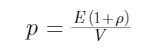it was necessary to measure E, the energy of the beam. This was done by a bolometric method in which the measurement of the energy was made to depend upon the resistance of the bolometer strip. Using the value of the energy thus obtained and 0.92  as the reflection coefficient of the silver surfaces, it was found that the pressure directly observed was about 20 per cent. lower than that computed from the Maxwell-Bartoli formula. After the publication of the paper, it was found that an error had been made in the measurell1ent of the resistance of the bolometer strip, owing to a fault in its construction. A new estimation of the resistance gave an energy value which brought the theoretical value of the pressure very close to that found by direct measurement. But another method of measuring the energy was adopted in the later experiments described in this paper.

In the number of the Annalen der Physik for Novel11ber, 1901, Professor Lebedew J published the results of a more varied series of measurements of radiation pressure than the early measurements of the present writers. The principal difference between the methods employed by him and by the writers for determining the pressure was that he used very thin metallic vanes surrounded by gas at extremely low pressures, thus following Maxwell's suggestion literally, while the writers used silvered glass vanes and worked at large gas pressures for which the gas action had been carefully and exhaustively studied and found to be negligibly small for short exposures. From our knowledge of the variation of gas action in different vacua, we feel sure that our method would not have been successful in high vacua because of the relatively large gas action. Professor Lebedew's own results, with blackened vanes of lower heat conductivity, show that his success in eliminating gas disturbance was due to the high heat conductivity of thin vanes rather than to the high vacua employed.

Professor Lebedew estimate of the accuracy of his work is such as to admit of possible errors of 20 per cent. in his final results. An analysis of Professor Lebedew's paper and comparison with our preliminary experiments seem to show that his accidental errors were larger than ours, but through the undiscovered false resistance in the bolometer our final results were somewhat farther from the theory than his. Either of the above researches would have been sufficient to establish the existence of a pressure due to radiation, but neither research offered, in our judgment, a satisfactory quantitative confirmation of the Maxwell-Bartoli theory.

By the way, the present author hereby mentions that in the article , Lebedew finally summarized his own experiment as follows:

1. The incident light beam exerts pressure on both the absorber and the reflector; this pan-dynamic effect has nothing to do with the known secondary Cruise force caused by warming and convection phenomena.
2. The pressure of these lights is proportional to the incident energy and has nothing to do with the color of the light,

3. Within the experimental error range, the pressure of these lights coincides in quantity with the kinetic energy of radiation calculated by Maxwell and Bartoli.

Therefore, the existence of the compressive force of Maxwell - Bartoli's rays has been proved through experiments

The author first puts forward the following concerns: The experimenters of these two experiments believed that the previous data from the Crookes radiometer light pressure experiment greatly exceeded the predicted value of formula (1). It was wrong. To cater to (1), they redesigned complex equipment and did very complex experiments. Their experiments were all subjectively preconceived by formula (1).

Do the above two experiments really measure the light pressure expressed by (1) or (1')? This is absolutely impossible. For example, in 1998, MW Evens, JP Vigier, S. Roy, G. Hunter, an important book on the basic theories of physics <The Enigmatic Photon: Volume 4: New Direction>  , this volume brings together a number of articles from leading in-depth review papers by experts. Wherein the electromagnetic radiation comprises a paper on a press of the optical published jointly by the Hungarian experts Pál R. MOLNáR, Tamás BORBéLY, Bulcsu FAJSZI , the paper demonstrated Lebedew proved only light pressure ratio, instead of an equalityHe suggested using contemporary laser and vacuum technology to make new measurements of light pressure.

Through the study of Lebedew experiment  and the experiment of E.F. Nichols and G.F. Hull , the author of this article believes that the judgment of the paper  is fully reasonable, not to mention that the Maxwell-Bartoli formula is not light pressure at all. Their experiments are just to cater to incorrect theories.

In fact, during this stage of exploration, the author found that no one in his scholar circle had personally seen this type of experiment, nor he had seen any report of repeating such an experiment.

The author believes that the experimental data of F. Zöllner in 1877 is simple and reliable. It is not an experiment to cater to any authoritative formula but is measured based on the operation of the radiometer. These data reveal the existence and magnitude of light pressure. This order of magnitude is much larger than that given by the Maxwell-Bartoli formula, and it also reflects the general rule that the measured light pressure is less than the “equivalent light pressure”.

The role of Crookes radiometer should not be underestimated. By further increasing the vacuum degree, reducing possible interference resistance, and appropriately increasing the intensity of irradiation to make other interference relatively small, the radiometer may be used for more accurate measurement of light pressure.

Through experiments with this kind of radiometer, the author discovered more effects of light on matter. For example, under low temperature and low radiation of the external environment, a while after the rotation caused by the light stops, the blades of the radiometer will rotate right and left several times repeatedly. See the following attached video: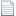DSCN2090_2_2_2.MP4

or

This phenomenon may reveal a possible heat radiation mechanism of metal surface materials, that is, the metal on the surface must first store the heat conducted from the internal material, and when the heat accumulates to a certain extent, it quickly releases heat through radiation. This possible mechanism determines that the heat radiation from the material with the metal surface be carried out discontinuously and sequentially in batches. This can explain the repeated alternation of the direction of rotation of the radiometer blades.

Obviously, many other changes in the state of matter are subjects that require more in-depth study.

V Concluding remarks

As mentioned in the introduction of M.W. Evens and other joint work <The Enigmatic Photon> , "This volume brings together several in-depth review papers from leading experts, establishing a fact that electrodynamics absolutely Not a fully understood theory".

Yes, the mysterious photon, you have too many secrets for humans to explore!

references:

 James Clerk Maxwell , " A Dynamical Theory of the Electromagnetic Field ",  Philosophical Transactions of the Royal Society of London 155 , 459–512 (1865).

 JC MAXWELL, A Treatise on Electricity and Magnetism (1St ed.), Vol. 2, Oxford, 1873.

 JC Maxwell, Lehrbuch der Elektricitiit und des Magnetismus. Deutsch von B. Weinstein, Berlin 1883.

 A. Bartoli, Ezner's Bep. d. Phys. 21. p. 198. 1884, übersetzt aus Nuovo Cimento 16. p. 195. 1883.

 P. Lebedew: Untersuchen uber die druckkrafte des Lichtes, Annalen der Physik 46, 432 (1901).

  Poynting, John Henry (1884) . "On the Transfer of Energy in the Electromagnetic Field". Philosophical Transactions of the Royal Society of London.  175 : 343–361.

   LD Landau, EM Lifshitz (1975).  The Classical Theory of Fields . Vol. 2 (4th ed.). Butterworth–Heinemann.  ISBN 978-0-7506-2768-9.

 Crookes, William, (1 January 1874).  "On Attraction and Repulsion Resulting from Radiation". Philosophical Transactions of the Royal Society of London.  164 : 501–527. doi:10.1098/rstl.1874.0015. S2CID 110306977 ..

 F. Zöllner, Pogg. Ann. 160. p. 154. 1877.

   James Clerk Maxwell,  On stresses in rarefied gases arising from inequalities of temperature , Royal Society Phil. Trans. (1879)

 Osborne Reynolds,    On certain dimensional properties of matter in the gaseous state , Royal Society Phil. Trans., Part 2, (1879)

  Nichols, E. F & Hull, GF The pressure due to the radiation,  The Astrophysical Journal , Vol.17 No.5, p.315-351 (1903)

 Myron Evans, Geoffrey Hunter, Jean-Pierre Vigier,  <The Enigmatic Photon:  Volume 4: New Directions>, Publisher: Kluwer Academic Publishers, SBN: 9780792348269, ISBN-10: 9780792348269 (1998)

https://wap.sciencenet.cn/blog-553379-1294399.html

全部精选博文导读

GMT+8, 2022-1-23 08:32